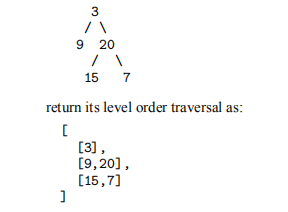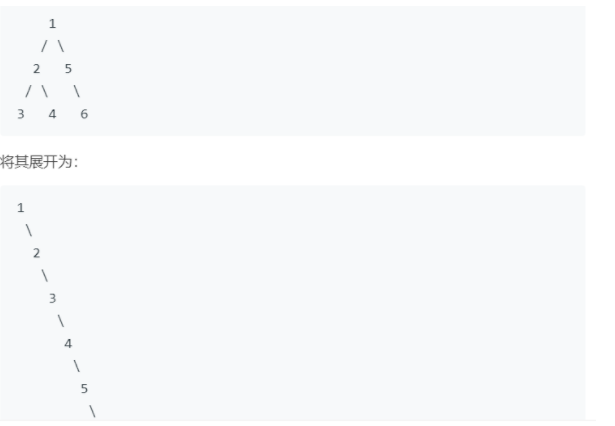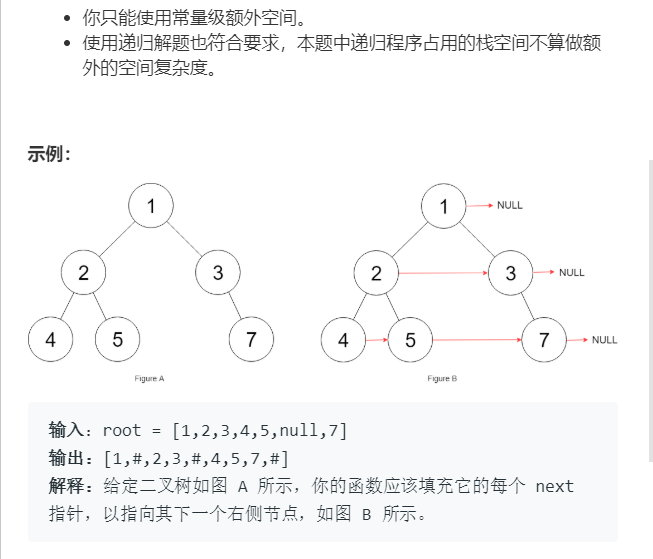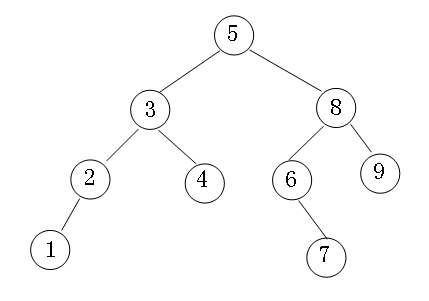• 其中的对于及基础的遍历，以及对于各种遍历情况下的衍生出来的也是层出不穷，今天就来记录在学习过程中遇到的一些基础的遍历过程中的算法题目，也是系列的第一讲。 注：以下所有代码皆使用Java进行编写 的各种...
引言
众说周知，树在算法领域算是一个比较大的分支结构。其中的对于树及基础的遍历，以及对于各种遍历情况下的衍生出来的也是层出不穷，今天就来记录在学习过程中遇到的一些基础的遍历过程中的算法题目，也是系列的第一讲。
注：以下所有代码皆使用Java进行编写
树的各种遍历：
首先我们需要了解一下数的各种遍历：分为先序，中序，后序，层序；
讲解：对于树的操作而言，由于在很多的情况下都是会回退到对上一级的访问，所以在对于树的算法中很多的情况在在遍历的过程中会常常使用到栈（先入后出），队列（先入先出），或者是直接只用到递归来进行解题，因为对于递归来说，在特定的时候，进行一个判断，就能够很好的回退到对上一层的操作上面。当然泛泛而谈也不是我们的特点，下面我来具体的讲解各种的遍历操作，以及在LeetCode上面，对于树的遍历的一部分题型。
树的数据结构：
  public class TreeNode {
int val;
TreeNode left;
TreeNode right;
TreeNode(int x) { val = x; }
}

前序遍历
先序：根->左子树->右子树；
思想：： 提及到先序遍历（这里我就不再画具体的图来进行解释什么是先序遍历）我们所有的序操作都是对于根节点来说，所以先序是先进性根节点，然后左子树，然后再右子树。此时想到前面所提及到的基础的使用方法。可以使用到栈来进行一个辅助，但是此时又出现了一个问题，对于栈来说是先进后出，我们想要实现访问完根节点能够对右子树进行输出，所以此时就不能够先把右子树放进去，先放左子树，然后再放右子树，就可以实现我们想要实现的操作。
代码展示：
import java.util.ArrayList;
import java.util.Stack;
public class Solution {
public ArrayList<Integer> preorderTraversal(TreeNode root) {
ArrayList <Integer> array=new ArrayList<Integer>();
Stack<TreeNode> stack=new Stack<TreeNode> ();
if(root==null)
return array;
stack.push(root);
while(!stack.isEmpty()){
TreeNode temp=stack.pop();
array.add(temp.val);
if(temp.right!=null){
stack.push(temp.right);
}
if(temp.left!=null)
stack.push(temp.left);
}
return array;
}
}

讲解：：首先我们要解决的问题：

使用到什么数据结构，这里也算是提醒大家，在树的操作中，不是栈就是队列，当然栈的操作会更多，也更加符合树的特性，然后就是使用链表来进行接收操作。
由于之前讲过，实现根->左->右,但是对于栈的操作却是要反而行之，由于每一个小的子树却也都是一课子树，所以在操作时候，要放在一个循环中进行执行。
使用一个pop出来的值，进行左右操作，就可以解决上一阶级的问题。

中序遍历
左->根->右
思想： 既然是先左子树，我们要解决的第一个问题就是说对于很多层的，我们首先要做的肯定就是使用一个while循环知道位于树的最底层的最左边的一个节点。然后在一些的节点不存在时候，适当进行pop依次得到上一层的节点的信息，再度进行另外一边节点的操作。
代码：
import java.util.*;
public class Solution {
public ArrayList<Integer> inorderTraversal(TreeNode root) {
ArrayList<Integer> array=new ArrayList<Integer>();
Stack<TreeNode> stack=new Stack<TreeNode>();
if(root==null) return array;
//  stack.push(root);
TreeNode node=root;
while(node!=null ||!stack.isEmpty()){
while(node!=null){
stack.push(node);
node=node.left;
}
TreeNode temp=stack.pop();
array.add(temp.val);
node=temp.right;
}
return array;
}
}

讲解：：如上我们可以看到的是，同先序遍历的大值思想是一致的，进行栈的判空是为了能够判断是否对整个树都进行扫描。同样先进入到最底端的左节点，然后不成功时候再度向上一级索引（这里就会使用到栈的pop()）。然后再循环操作即可。
后序遍历
左->右->根
思路: 和先序遍历的情况正好相反，此时我们可以设想一下，在先序中，我们对于根来说，根在前，然后是左右。此时的情况是，根在后，然后左右在前。此时我们可以转换思路，既然现在情况是 根在最后，不如我们照常来进行遍历，最后都压在一个栈中，然后，统一对栈进行pop() 时候，即可完成我们想要的需求，但是此时有一个问题，队友左右根的出栈，就是，根右左的压栈，此时，右先左的前面，所以此时，先遍历左子树，再度进行右子树。
public class Solution {
public ArrayList<Integer> postorderTraversal(TreeNode root) {
ArrayList<Integer> array=new ArrayList<Integer>();
Stack<TreeNode> stack=new Stack<TreeNode>();
Stack<TreeNode> stacks=new Stack<TreeNode>();
if(root==null)
return array;
stack.push(root);
while(!stack.isEmpty()){
TreeNode temp =stack.pop();
stacks.push(temp);
if(temp.left!=null){
stack.push(temp.left);
}
if(temp.right!=null)
stack.push(temp.right);
}
while(!stacks.isEmpty()){
TreeNode c=stacks.pop();
array.add(c.val);
}
return array;

}
}

层序遍历 使用到了 队列
思路： 层序遍历，顾名思义，就是对于树的一层一层组成遍历，不同于之前讲到的遍历而言，逐层遍历，不需要再次进行左右的切换，此时也不需要用到栈的那种特性，但是此时思考的问题是：“如何在访问完一个根节点的左右子树之后，再度返回到由右子树组成的一个小的子树，此时就使用到了队列的特性、先访问的左子树，也是先进入队列，然后对于先进入的再次先进行访问，也是满足我们的需求–从左到右的依次。”
import java.util.*;
public class Solution {
public ArrayList<ArrayList<Integer>> levelOrder(TreeNode root) {
Queue<TreeNode> queue=new LinkedList<TreeNode>();
ArrayList<ArrayList<Integer>> arrays=new ArrayList<ArrayList<Integer>>();
ArrayList<Integer> array=new ArrayList<Integer>();
if(root==null)return arrays;
queue.offer(root);
// array.add(root.val); 如何进行表示表示他们是在同一层 是个问题的关键所在  可以利用for循环来实现判断是否还存在数据供我们访问，是一个经典所在。
while(queue.size()!=0){
int len=queue.size();
for(int i=0;i<len;i++){
TreeNode temp=queue.poll();
if(temp.left!=null){
queue.add(temp.left);
}
if(temp.right!=null){
queue.add(temp.right);
}
array.add(temp.val);
}
arrays.add(array);
array=new ArrayList<Integer>();
}
return arrays;
}
}

变形讲解
102-Binary Tree Level Order Traversal
实现效果如下：思路：自是对于层序遍历的一个小小的变形，在最开始的所有的遍历中，输出的都是一个**（ArrayList）链表**。但是此时我们需要输入的不再是简简单单的链表，而是以数组为泛型的数组。这里需要进行讲解一下：我们可以先行创建一个数组，由于存放的是每一行的元素，这个不难实现，下面，我们需要做到的是，将这每一行的数组再加入到以一个数组为泛型的数组中。让我举个栗子：
栗子：
如下所示，在进行泛型数组的添加时候，对于相同名字的数组在添加时候会进行覆盖的情况，所以完成一次对以数组为泛型的数组的add以后，要对数组类型进行一次新的new（创建一个全新的对象，再次进行添加操作）
 @Test
public static void main(String[] args) {
ArrayList<Integer> arrayList=new ArrayList<Integer>();
ArrayList<ArrayList<Integer>> arrayLists=new ArrayList<ArrayList<Integer>>();
arrayList.add(1);
arrayList.add(2);
System.out.println(arrayList);
arrayLists.add(arrayList);
System.out.println(arrayLists);
arrayList=new ArrayList<>();
arrayList.add(3);
arrayList.add(4);
System.out.println(arrayList);
arrayLists.add(arrayList);
System.out.println(arrayLists);
}

打印结果如下：
[1, 2]
[[1, 2]]
[3, 4]
[[1, 2], [3, 4]]

代码：
class Solution {
public List<List<Integer>> levelOrder(TreeNode root) {
List<List<Integer>> levels = new ArrayList<List<Integer>>();
if (root == null) return levels;
Queue<TreeNode> queue = new LinkedList<TreeNode>();
queue.add(root);
int level = 0;
while ( !queue.isEmpty() ) {
levels.add(new ArrayList<Integer>());
int level_length = queue.size();
for(int i = 0; i < level_length; ++i) {
TreeNode node = queue.remove();
levels.get(level).add(node.val);
if (node.left != null) queue.add(node.left);
if (node.right != null) queue.add(node.right);
}
level++;
}
return levels;
}
}

100- same-tree
思路：判断一个数是否完全和另外一个数是相等的，也是要不断得实现对数的遍历。下面提供两种方法解决。
递归：重点是不同情况的判断
public class Solution {
public boolean isSameTree(TreeNode p, TreeNode q) {
return firstList(p,q);
}
private boolean firstList(TreeNode root1,TreeNode root2){
if(root1==null &&root2==null)
return true;
if(root1==null || root2==null)
return false;
if(root1.val!=root2.val)
return false;
return firstList(root1.left,root2.left)&&firstList(root1.right,root2.right);
}
}

迭代：重点是对栈的利用+各种不成立情况判断。
import java.util.*;
public class Solution {
public boolean isSameTree(TreeNode p, TreeNode q) {
Stack<TreeNode> stack=new Stack<TreeNode>();
stack.push(p);
stack.push(q);
while(!stack.isEmpty()){
p=stack.pop();
q=stack.pop();
if(p==null && q==null) continue;
if(p==null || q==null) return false;
if(p.val!= q.val) return false;
stack.push(p.left);
stack.push(q.left);
stack.push(p.right);
stack.push(q.right);
}
return true;
}
}

110 Balance-binary-tree
判断给定的一个二叉树是不是平衡二叉树;
平衡二叉树：每一个结点的左右两个子树的高度差不超过1.
思路: 对于这种问题，之前我们也讲过试想一下，判断这种问题的关键在于得出一个二叉树的左右节点的高度，然后进行判断最高的高度减去最低的高度的值是否是大于1的，那么如何得到一棵二叉树的高度，此时就可以使用到递归的算法，不断递归进入左右子树，对其的高度加一，再使用一个max函数和min函数进行减法运行即可。
public class Solution {
boolean flag=false;
public boolean isBalanced(TreeNode root) {
depth(root);
return !flag;
}
private int depth(TreeNode t){
if(flag ||t==null)
return 0;
int left=depth(t.left);
int right=depth(t.right);
if(Math.max(left,right)-Math.min(left,right)>1)
{
flag=true;
}
return 1+Math.max(left,right);
}
}

114 Flatten Binary Tree to Linked List
将一个二叉树转换成为一个链表类型的二叉树（要求使用原地转换）思路： 其实这道题目可以使用最基础的先序遍历来解决，每一次先序遍历的层次都是从左到右的输出，这个时候将其接在右子树上即可，但是这里有一个小小的问题，对于c++来说栈的操作有一个 top() 函数可以实现对栈顶元素进行访问。但是对于java并没有这个函数，所以想要使用这个方法时候，还需要再度创建多于的栈用来存放已经遍历出来的元素，再次存放到里面，就是多于的空间消耗。于是就有了一下的解法，思路也是比较简单。
public void flatten(TreeNode root) {
while (root != null) {
//左子树为 null，直接考虑下一个节点
if (root.left == null) {
root = root.right;
} else {
// 找左子树最右边的节点
TreeNode pre = root.left;
while (pre.right != null) {
pre = pre.right;
}
//将原来的右子树接到左子树的最右边节点
pre.right = root.right;
// 将左子树插入到右子树的地方
root.right = root.left;
root.left = null;
// 考虑下一个节点
root = root.right;
}
}
}

117-Populating Next Right Pointers in Each Node II思路 ：这个题目有使用到 pummy指针，在这个题目之前，还有一个是完全二叉树，这里不再展示出来，使用简单的递归，还是比较容易实现的。但是对于不是完全二叉树的实现，可能就需要用到不同的思想。这里的pummy指针就是比较好的思想：一个空节点在开始时候指向当前层，定义一个pre前驱指针指向当前层的下一层，进行操作，在完成以后，此时的dummy指针的next指针已经发生了变化，指向了当前层的下一次，此时再次开始新一轮的操作，于此往复。
代码：
public class Solution {
public void connect(TreeLinkNode root) {
if(root==null)
return;
TreeLinkNode node=new TreeLinkNode (0);
TreeLinkNode cur;
TreeLinkNode pre=node;
for(cur=root;cur!=null;cur=cur.next){
if(cur.left!=null){
pre.next=cur.left;
pre=pre.next;
}
if(cur.right!=null){
pre.next=cur.right;
pre=pre.next;
}
}
connect(node.next);
}
}

结语: 以上就是关于在遍历阶段所出现的一些算法题目，或多或少都有一些遍历的影子。写这一系列博客的主要原因是，觉得泛泛的刷力扣不能达到总结的效果，打算把系列的题目总结起来，也方便在以后面试的复习中，能够很快找到要点。


展开全文• 中序遍历非递归算法 1)后置订单算法 (1) Algorithm for Postorder) In this traversal first, traverse the leftmost subtree at the external node then visit the root node and lastly traverse the right ...
树中序遍历非递归算法 1)后置订单算法 (1) Algorithm for Postorder)
In this traversal first, traverse the leftmost subtree at the external node then visit the root node and lastly traverse the right subtree starting at the left external node.
在此遍历中，首先遍历外部节点上最左侧的子树，然后访问根节点，最后遍历从左侧外部节点开始的右侧子树。
POSTORD(INFO, LEFT, RIGHT, ROOT)
Step-1 [Push NULL onto STACK and initialize PTR]
Set TOP=1, STACK=NULL and PTR=ROOT.

Step-2 [Push left-most path onto STACK] repeat step 3 to 5 while PTR not equal NULL.

Step-3 set TOP=TOP+1 and STACK[TOP]=PTR. [Pushes PTR on STACK].

Step-4 if RIGHT[PTR] not equal NULL then [push on STACK.]
Set TOP=TOP+1 and STACK[TOP]= RIGHT[PTR]
[End of if structure]

Step-5 set PTR = LEFT[PTR].[Update pointer PTR]
[End of step 2 loop].

Step-6 set PTR= STACK[TOP] and TOP=TOP-1.
[Pops node from STACK].

Step-7 Repeat while PTR>0;
Apply PROCESS TO INFO[PTR].
Set PTR= STACK[TOP] and TOP= TOP-1.
[Pops node from STACK]
[End of loop]

Step-8 if PTR<0 then:
Set PTR= -PTR
Go to step 2
[End of if structure]

Step-9 Exit.

2)排序算法 (2) Algorithm for Inorder)
In this traversal first traverse, the root node then traverses the left subtree of the external node and lastly traverse the right subtree of the external node.
在此遍历中，根节点然后遍历外部节点的左子树，最后遍历外部节点的右子树。
INORD( INFO, LEFT, RIGHT, ROOT)
Step-1 [Push NULL onto STACK and initialize PTR;]
Set TOP=1 STACK= NULL and PTR=ROOT.

Step-2 Repeat while PTR not equal NULL[Pushes left most path onto STACK].
a)	Set TOP=TOP+1 and STACK[TOP]=PTR [saves node]
b)	Set PTR=LEFT[PTR] [updates PTR]
[End of loop]

Step-3  set PTR= STACK[TOP and TOP=TOP-1.[pops node from STACK].

Step-4 Repeat step 5 to 7 while PTR is not equal to Null; [backtracking].

Step-5 Apply PROCESS to INFO[PTR],

Step-6 [RIGHT child?] if RIGHT[PTR] is not equal NULL then.
a)	Set PTR=RIGHT[PTR]
b)	Go to step 3.[End of if structure.]

Step-7 set PTR= STACK[TOP] and TOP=TOP-1. [pops node]
[End of step 4 loop]

Step-8 Exit.

3)预购算法 (3) Algorithm for Preorder)
In this traversal first, traverse all the left external node starting with the leftmost subtree which is then followed by bubble-up all the external node and then traverse the right subtree starting at the left external node which is the followed by bubble-up all the internal nodes and lastly visit the root node.
在此遍历中，首先遍历所有左外部节点，从最左边的子树开始，然后遍历所有外部节点，然后遍历右子树，从左侧外部节点开始，随后遍历所有内部节点，最后访问根节点。
PREORDER(  INFO, LEFT,RIGHT, ROOT)
Step-1 [initially push NULL onto stack and initialize PTR.]
Set TOP=1 STACK=NULL and PTR= ROOT.

Step-2 Repeat step 3 to 5 while PTR not equal NULL.

Step-3 Apply PROCESS to INFO[PTR].

Step-4 [RIGHT child?]
If RIGHT[PTR] not equal NULL then [PUSH on STACK]
Set TOP= TOP+1 and STACK[TOP]= RIGHT[PTR]
[End of if structure.]

Step-5 [LEFT child?]
If LEFT[PTR] not equal NULL then
Set PTR= LEFT[PTR].
Else [pop from STACK;]
Set PTR= STACK[TOP] and TOP=TOP+!;
[End of if structure]
[End of step 2 loop]

Step-6 Exit.


翻译自: https://www.includehelp.com/algorithms/non-recursive-tree-traversal-algorithm.aspx树中序遍历非递归算法
展开全文• CLRS 12.1-3：来源：...给出一个非递归的中序树遍历算法。(提示：有两种方法，在较容易的方法中，可以采用栈作为辅助数据结构；在较为复杂的方法中，不采用栈结构，但假设可以测试两个指针是否相等。)
CLRS 12.1-3：

来源：http://www.cnblogs.com/shuaiwhu/archive/2011/04/20/2065055.html
给出一个非递归的中序树遍历算法。(提示：有两种方法，在较容易的方法中，可以采用栈作为辅助数据结构；在较为复杂的方法中，不采用栈结构，但假设可以测试两个指针是否相等。)

算法思想：

1.采用栈的话，先寻找最左边的节点，把经过的节点都存入栈中，第一个被弹出来的为最左节点，那么访问其右子树，对右子树也像前面一样遍历，整个流程跟递归一样。

2.不采用栈的话，先是访问最左节点，然后访问其右子树，然后回溯到最左节点的父节点，不断重复这个过程，思路还是一样。这里参考了重剑无锋的http://blog.csdn.net/kofsky/archive/2008/09/05/2886453.aspx

构造的树的树如下：#include <iostream>
#include <time.h>
usingnamespace std;
class Node
{
public:
int data;
Node* left;
Node* right;
Node* parent;
bool  visited;
//Node(){}
Node(int d);
Node(int d, Node* l, Node* r);
};
class BinaryTree
{
public:
Node* root;
BinaryTree(Node* r);
//递归实现中序遍历
void recurse_in_order_visit(Node* root);
//非递归用栈实现中序遍历
void non_recurse_using_stack_in_order_visit(Node* root);
//非递归且不用栈实现中序遍历
void non_recurse_non_stack_in_order_visit(Node* root);
enum TRAVESAL_STATE
{
LEFT_NOT_TRAVERS,//左子树未遍历
LEFT_TRAVERSED,//左子树已遍历(包括左子树为空)
RIGHT_TRAVERSED//右子树已遍历(右子树为空)
};
};
int main()
{
Node* node1 =new Node(1, NULL, NULL);
Node* node2 =new Node(2, node1, NULL);
Node* node3 =new Node(4, NULL, NULL);
Node* node4 =new Node(3, node2, node3);
Node* node5 =new Node(7, NULL, NULL);
Node* node6 =new Node(6, NULL, node5);
Node* node7 =new Node(9, NULL, NULL);
Node* node8 =new Node(8, node6, node7);
Node* root =new Node(5, node4, node8);
BinaryTree* binary_tree =new BinaryTree(root);
cout<<"递归中序遍历的结果："<<endl;
binary_tree->recurse_in_order_visit(binary_tree->root);
cout<<endl;
cout<<"非递归用栈中序遍历的结果："<<endl;
binary_tree->non_recurse_using_stack_in_order_visit(binary_tree->root);
cout<<endl;
//若使用非递归且不用栈来进行中序遍历，则需要parent指针作为辅助
node1->parent = node2;
node2->parent = node4;
node3->parent = node4;
node5->parent = node6;
node6->parent = node8;
node7->parent = node8;
node4->parent = root;
node8->parent = root;
cout<<"非递归且不用栈中序遍历的结果："<<endl;
binary_tree->non_recurse_non_stack_in_order_visit(binary_tree->root);
cout<<endl;
return0;
}

Node::Node(int d)
{
data = d;
parent = left = right = NULL;
visited =false;
}

Node::Node(int d, Node* l, Node* r)
{
data = d;
left = l;
right = r;
parent = NULL;
visited =false;
}

BinaryTree::BinaryTree(Node* r)
{
root = r;
}

//递归实现中序遍历
void BinaryTree::recurse_in_order_visit(Node* root)
{
if(root != NULL)
{
recurse_in_order_visit(root->left);
printf("%d\t", root->data);
recurse_in_order_visit(root->right);
}
}

//非递归用栈实现中序遍历
void BinaryTree::non_recurse_using_stack_in_order_visit(Node* root)
{
Node* stack;
int top =-1;
while(root != NULL || top >-1)
{
while(root != NULL)
{
stack[++top] = root;
root = root->left;
}
if(top >-1)
{
root = stack[top--];
printf("%d\t", root->data);
root = root->right;
}
}
}

//非递归且不用栈实现中序遍历
void BinaryTree::non_recurse_non_stack_in_order_visit(Node* root)
{
while ( root != NULL )
{
while ( root->left != NULL &&!root->left->visited )
{
//一路向左
root = root->left;
}
if ( !root->visited )
{
cout<<root->data<<"\t";
root->visited=true;
}
if ( root->right != NULL &&!root->right->visited )
{
//右子树
root = root->right;
}
else
{
//回溯至parent节点
root = root->parent;
}
}
}
展开全文• 树遍历算法的应用 1、求树的深度的算法： int TreeDepth(CSTree T) { if(!T) return 0; else { h1 = TreeDepth( T->firstchild ); h2 = TreeDepth( T->nextsibling); return(max(h1...
树遍历算法的应用1、求树的深度的算法：int TreeDepth(CSTree T) {  if(!T) return 0;  else {     h1 = TreeDepth( T->firstchild );     h2 = TreeDepth( T->nextsibling);     return(max(h1+1, h2));   }} // TreeDepth2、输出树中所有从根到叶子的路径的算法:void AllPath( Bitree T, Stack& S ) {// <1> 输出二叉树中所有从根到叶子的路径 if (T) { Push( S, T->data ); if (!T->lchild && !T->rchild ) PrintStack(S); else {    AllPath( T-> lchild, S );    AllPath( T-> rchild, S );   } Pop(S); }} // AllPathvoid OutPath( Bitree T, Stack& S ) {// <2> 输出树中所有从根到叶子的路径while ( !T ) {  Push(s, T->data );  if ( !T->firstchild ) Printstack(s);  else OutPath( T->firstchild, s );  Pop(s);  T = T->nextsibling; } // while} // OutPath
展开全文• 在遍历儿叉时，常常使用的是递归遍历，或者是借助于栈来迭代，在遍历过程中，每个节点仅访问一次，所以这样遍历的时间复杂度为O(n)，空间复杂度为O(n)，并且递归的算法易于理解和实现，二叉树的递归遍历算法代码...
• 建立一棵二叉链表，分别输出此先根、中根和后根遍历序列 将上题编程，实现哈夫曼的构建和哈夫曼编码的设计
• java算法遍历 在计算机科学中，“”是一种广泛使用的抽象数据类型（ADT）或实现此ADT的数据结构，它模拟分层结构，其根值和带有父节点的子级子树表示为一组链接节点。 可以将数据结构递归定义为节点...数据结构 编程语言 c++ python
• 在计算机科学中，是广泛使用的抽象数据类型（ADT）或实现此ADT的数据结构，它模拟分层结构，其根值和带有父节点的子级子树表示为一组链接节点。 可以将数据结构递归定义为节点的集合（从根节点开始），其中每...
• int TreeDepth(CSTree T) { if(!T) return 0; else{ h1 = TreeDepth(T->firstchild); h2 = TreeDepth(T->nextsibling); return(max(h1+1,h2)); } }数据结构
• void AllPath(Bitree T, Stack &S)//输出二叉上从根到所有叶子结点的路径 { if(T) { Push(S,T->data); if(!T->Left&&!T->Right)//如果左指针和右指针同时为空，才说明该节点为叶子节点 PrintStack(S); ...数据结构
•python
• 输出二叉树中的结点并无次序要求，因此可用三种遍历算法中的任何一种完成，只需将访问操作具体变为输出操作即可。下面给出采用先序遍历实现的算法。 【算法描述】 void PreOrder(BiTree root) /* 先序遍历输出二叉树...
• 主要介绍了JavaScript实现遍历算法,结合实例形式分析了javascript针对结构的广度优先遍历与深度优先遍历实现方法,需要的朋友可以参考下
• 一种基于GPU的KD光线遍历算法，兰青，，针对KD加速的光线跟踪算法，实现了一种基于GPU的进入点搜索遍历算法。通过搜索和预设光束进入场景的起始节点，减少一次光线的遍�
• 重庆交通大学信息科学与工程学院 综合性设计性实验报告 班 级 物联网1501班 姓名 学号 李怿欣 631507030101 实验项目名称 遍历算法 实验项目性质 综 合 性 实验所属课程 算法与数据结构 实验室(中心) 语音楼801...
• 主要介绍了JavaScript的深度优先遍历和广度优先遍历算法,结合实例形式分析了JavaScript的深度优先遍历、广度优先遍历递归与非递归相关实现技巧,需要的朋友可以参考下
• 主要介绍了JavaScript实现多叉的递归遍历和非递归遍历算法,结合实例形式详细分析了JavaScript多叉针对json节点的递归与非递归遍历相关操作技巧,需要的朋友可以参考下
• 通用遍历算法，适合所有的 /*的遍历，通用算法*/ #include typedef struct E_node{ char data; struct E_node *child[M];//M为的度 }E_NODE; void pre_print(E_NODE *T)//前序遍历M次 { int i; if...
• 这几种遍历方法，其时间复杂度都为O(n)，而空间复杂度递归和栈为O(h)，线索需要额外的标识位来表明是线索还是节点指针，空间复杂度为O(n)，当节点数量非常大时，高h = log(n)仍然较大，有没有其他的遍历算法，其...数据结构
• 树遍历的口诀 树的递归遍历代码 树的先序遍历 树的中序遍历 树的后序遍历 递归遍历思想 树的非递归遍历 树的先序非递归遍历 先序遍历运行结果 树的中序非递归遍历 中序遍历运行结果 树的后序非递归遍历 树的遍历 ...数据结构
• 给出的自下而上，自右到左的层次遍历算法 算法实现 void LevelOrder(BiTree T){ InitQueue(Q); InitStack(S); BiTree p; EnQueue(Q, T); while(!IsEmpty(Q)){ //自上而下，自左往右遍历 DeQueue(Q, p); ...stack 数据结构...